Posted on: Monday, December 16, 2019 by Rajiv Popat

I did a talk in one of the Microsoft Events a couple of years ago where I took the audience back to basics using C# and the folks seemed to love it and gave it a five star rating. Simple things like function pointers, delegates, basic algorithms and data structures. I think everyone should recap at-least one of these topics every week and this series is my humble attempt to share what I recap with you. Today I'm recapping Bubble Sort.

Bubble Sort

Bubble sort is by far one of the simplest sorting algorithms. Let's assume that if you have 5 numbers in an array and you need to sort these numbers from low to high. Let's assume this is what the initial state of the array looks like: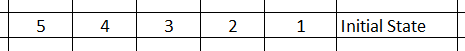Assuming you are sorting in ascending order, the goal is to run a for loop through every element in the array, using a simple for-loop and check if the number is larger than the number next to it. If the number is larger, swap it with the number on the right.

In the above example, the for-loop begins with 5. Because 5 is larger than 4, 4 and 5 are swapped. And then you compare 5 with 3. Basically here is how every iteration of the for loop looks like: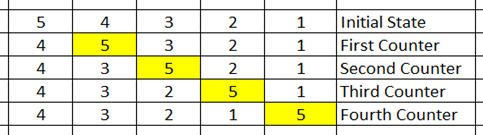Once the for loop completes you are left with: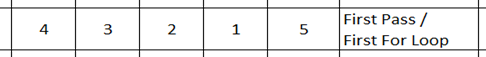One round of the for-loop execution is what we call the first pass on the array.

From the above image you can clearly see that if I do three more passes (i.e put the for-loop inside another for-loop) the array would be sorted and the right numbers would 'bubble up' to the right places. Here is what the second pass looks like: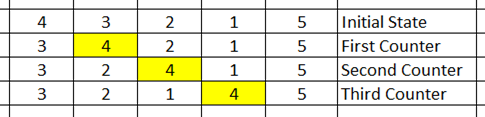From the above diagram it is intuitively evident that if you do this for three more passes this is what the outcome the third pass would look like: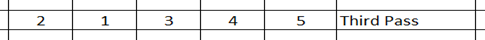And the fourth pass would look like this: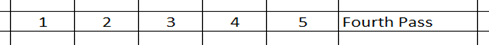So basically if you see each pass as a for-loop and 4 (N elements i.e. Len(array) - 1) passes as another loop, we can easily translate the above logic into code: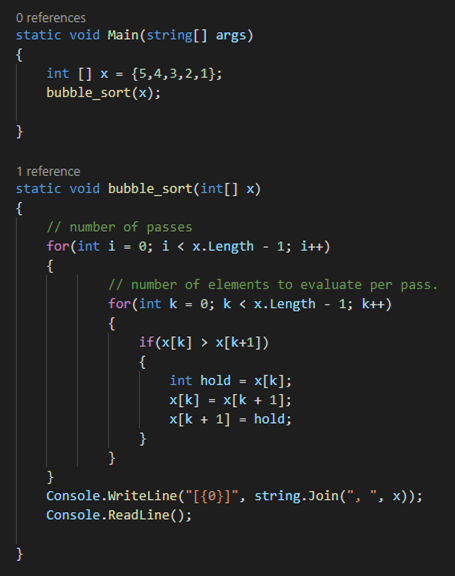In the above code, the inner for-loop is basically a single pass where we run through each element in an array and swap values if the value of the element is higher than the value it's next element. The outer for loops is number of passes.

When I wrote the example and published the blog, Steve was kind enough the point out in the comments section of this post that the algorithm wasn't fully optimized and than it was doing way more iterations than it really needed. And he is absolutely correct. In the above example we have two loops and both are iterating through the entire array. However if you notice in the above theory after the first pass, the largest number would have essentially moved to the extreme right of the array:What this means is the second pass can actually ignore the last element of the array when it iterates. The third pass can ignore the last two elements and so on. This means that the second loop can essentially be further optimized and doesn't have to iterate the highest numbers which have already been moved to the extreme right of the array. The optimized version of the code looks like this: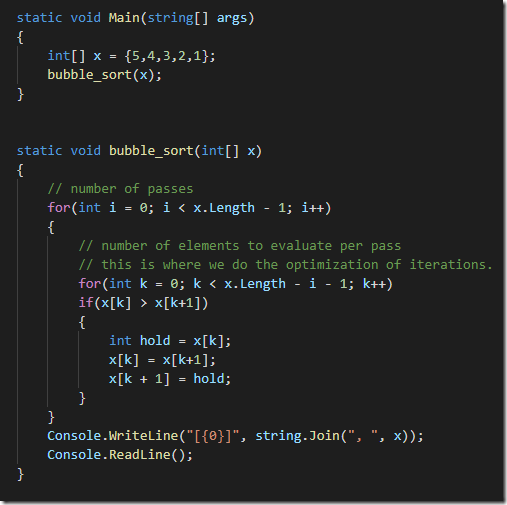If you want a slightly different way of doing the same thing you can also look at Steve's code in the comments of this post. Special thanks to him for pointing out the optimization which I had completely forgotten, which is why revisiting these topics every now and then often helps!

Bubble sort is not super efficient when it comes to it's time complexity, and there is hardly anywhere we hand code sorting, but the algorithm itself is something that gets thrown around a lot in discussions so it's good to know what bubble sorting is all about.

posted on Monday, December 16, 2019 10:52:43 AM UTC by Rajiv PopatComments  Trackback